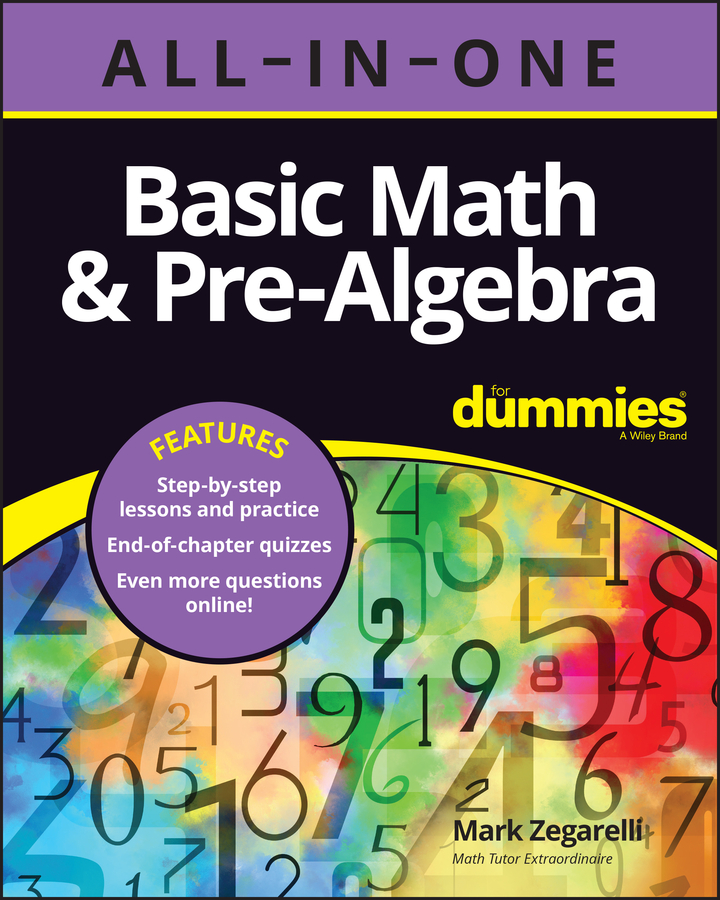##### Basic Math & Pre-Algebra All-in-One For Dummies (+ Chapter Quizzes Online)When you write out a word equation, you have the facts you need in a form you can use to find the solution. You can often solve the problem by plugging numbers from one word equation into another. The following examples show you how to use word equations to solve three problems.

## Example 1: addition and subtraction

Some problems involve simple addition or subtraction. Here’s an example:

Bobo is spinning five fewer plates than Nunu (Bobo dropped a few). Nunu is spinning 17 plates. Altogether, how many plates are Bobo and Nunu spinning?

From reading the problem, you know the following:

Nunu = 17

Bobo + 5 = Nunu

Plugging in the information gives you the following:

Bobo + 5 = Nunu 17

If you see how many plates Bobo is spinning, feel free to jump ahead. If not, here’s how you rewrite the addition equation as a subtraction equation:

Bobo = 17 – 5 = 12

The problem wants you to find out how many plates the two clowns are spinning together. That is, you need to find out the following:

Bobo + Nunu = ?

Just plug in the numbers, substituting 12 for Bobo and 17 for Nunu:

Bobo 12 + Nunu 17 = 29

So Bobo and Nunu are spinning 29 plates.

## Example 2: multiplication and division

At times, a problem may note relationships that require you to use multiplication or division. Here’s an example:

The height of a house is half as long as its width, and the width of the house is 80 feet. How tall is the house?

From the word equation, you know the following:

Width = 80

Height = Width ÷ 2

You can plug in information as follows, substituting 80 for the word width:

Height = Width 80 ÷ 2 = 40

So you know that the height of the house is 40 feet.

## Example 3: multiple equations

Pay careful attention to what the question is asking. You may have to set up more than one equation. Here’s an example:

The express train is moving three times faster than the local train. If the local train is going 25 miles per hour, what’s the difference in speed between the express train and the local train?

From the word equation, you know the following:

Local = 25

Express = 3 · Local

So plug in the information you need:

Express = 3 · 25 Local = 75

In this problem, the question at the end asks you to find the difference in speed between the express train and the local train. Finding the difference between two numbers is subtraction, so here’s what you want to find:

Express – Local = ?

You can get what you need to know by plugging in the information you’ve already found:

Express 75 – Local 25 = 50

Therefore, the difference in speed between the express train and the local train is 50 miles per hour.